# Wolfram LaTeX Character-Level Language Model V1

Generate LaTeX code

This language model is based on a simple stack of gated recurrent layers. It was trained by Wolfram Research in 2018 using teacher forcing on sequences of length 100.

Number of layers: 7 | Parameter count: 7,896,330 | Trained size: 32 MB |

## Training Set Information

• Internal Wolfram training set, consisting of over 5 GB of LaTeX code scraped from 150,000 articles from arXiv.

## Examples

### Resource retrieval

Get the pre-trained network:

 In:=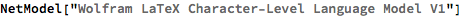Out=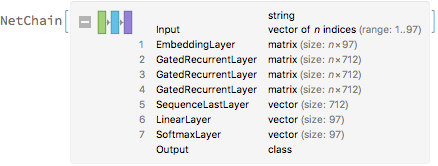### Basic usage

Predict the next character of a given sequence:

 In:=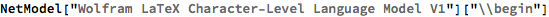Out=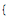Get the top 15 probabilities:

 In:=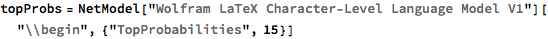Out=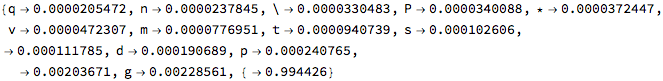Plot the top 15 probabilities:

 In:=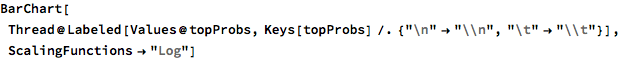Out=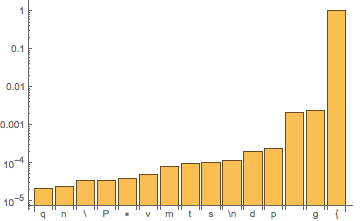### Generation

Generate text efficiently with NetStateObject. A built-in option for temperature sampling is available in Wolfram Language 12.0, while it has to be implemented explicitly in earlier versions.

 In:=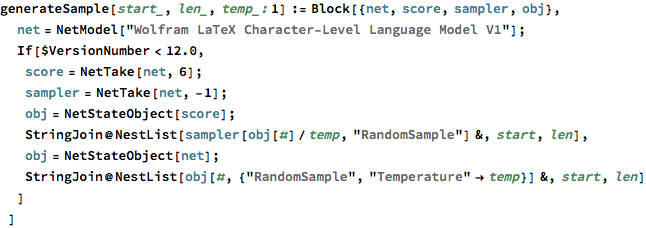Generate for 100 steps using “\begin” as an initial string:

 In:=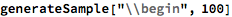Out=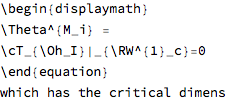The third optional argument is a “temperature” parameter that scales the input to the final softmax. A high temperature flattens the distribution from which characters are sampled, increasing the probability of extracting less likely characters:

 In:=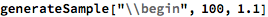Out=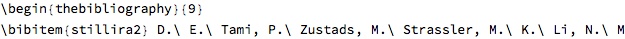Decreasing the temperature sharpens the peaks of the sampling distribution, further decreasing the probability of extracting less likely characters:

 In:=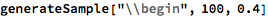Out=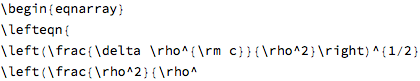Very high temperature settings are equivalent to random sampling:

 In:=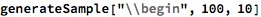Out=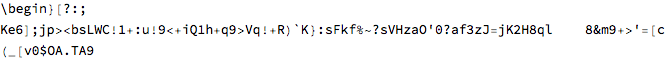Very low temperature settings are equivalent to always picking the character with maximum probability. It is typical for sampling to “get stuck in a loop”:

 In:=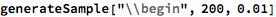Out=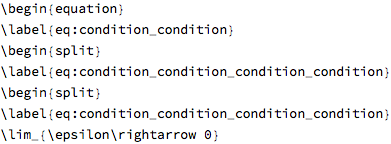### Inspection of predictions

Define a function that takes a string and guesses the next character as it reads, showing the predictions in a grid. The input string is shown on top, while the top 5 predictions are aligned below each character, starting from more likely guesses. For each prediction, the intensity of the color is proportional to the probability:

 In:=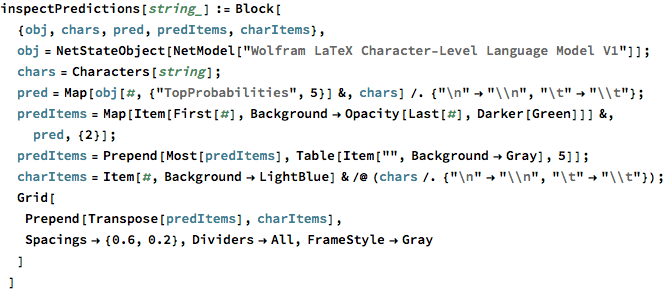In:=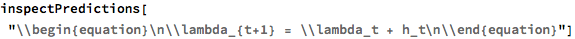Out=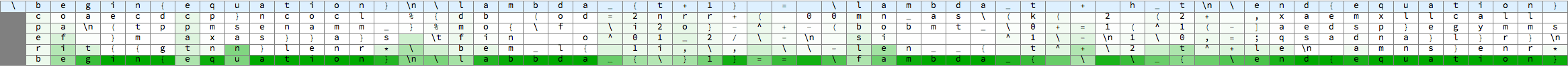### Net information

Inspect the sizes of all arrays in the net:

 In:=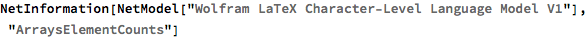Out=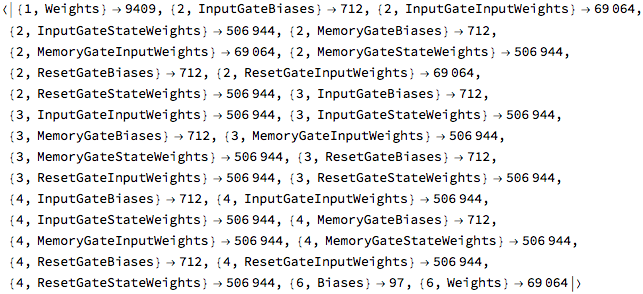Obtain the total number of parameters:

 In:=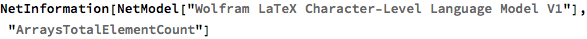Out=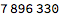Obtain the layer type counts:

 In:=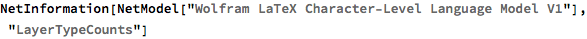Out=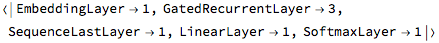Display the summary graphic:

 In:=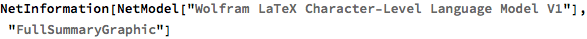Out=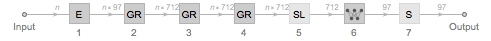### Export to MXNet

Export the net into a format that can be opened in MXNet:

 In:=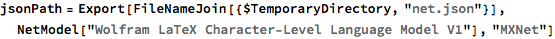Out=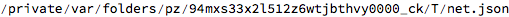Export also creates a net.params file containing parameters:

 In:=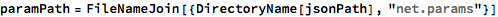Out=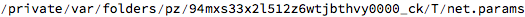Get the size of the parameter file:

 In:=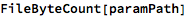Out=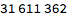The size is similar to the byte count of the resource object:

 In:=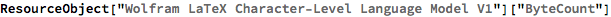Out=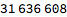## Requirements

Wolfram Language 11.3 (March 2018) or above

## Reference

• Wolfram Research (2018)